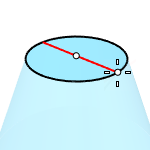# MeshTruncatedCone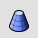Mesh

Polygon Mesh Primitives >

Truncated Cone

The MeshTruncatedCone command draws a mesh truncated cone.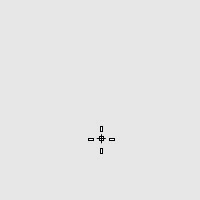#### Steps

1. Draw the base circle.

2. Pick a location or type a number to set the height.

The positive direction of the height is different based on the selected DirectionConstraint option.

3. Draw the end circle.

Base circle options

### DirectionConstraint

Direction constraints restrict the direction of the truncated cone.

##### None

Pick or type a number to set the height.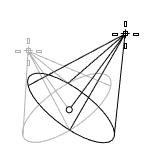##### Vertical

Creates a truncated cone perpendicular to the construction plane.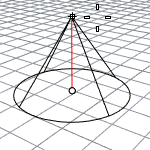• The CPlane +Z direction is the positive direction when you type a number to set the height.
##### AroundCurve

Draws the base circle perpendicular to the picked point on a curve. The center line of the truncated cone will be tangent to the curve.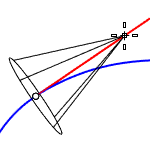• The curve direction is the positive direction when you type a number to set the height.

### Solid

Caps the top and bottom openings or leave them open.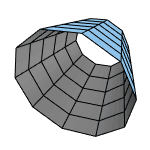Draws the base circle by picking the center point and a radius point.### 2Point

Draws the base circle from two opposite points.### 3Point

Draws the base circle through three points.### Tangent

Draws the base circle tangent to one, two, or three curves.### FitPoints

Draws the base circle by fitting to selected points, control points, or mesh vertices.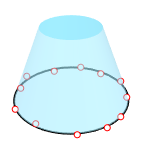### VerticalFaces

The number of faces in the vertical direction.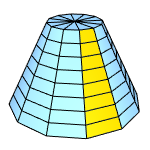### AroundFaces

The number of faces around the circumference.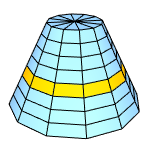End circle options

Pick a location or enter a number to set the end circle radius.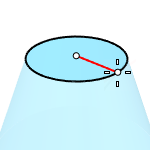### Diameter

Pick a location or enter a number to set the end circle diameter.